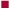# rule of nines

rule of nines
a method of estimating the extent of body surface that has been burned in an adult; the body surface is divided into sections of 9 per cent or multiples of 9 per cent. See accompanying table. For children the Lund-Browder classification provides a more accurate estimate.Rule of nines.

Medical dictionary. 2011.

### Look at other dictionaries:

• Rule of nines (mathematics) — The rule of nines, in mathematics, is a divisibility rule for the divisor 9. It is notable because it illustrates some interesting properties of modular arithmetic, and its proof is derived from that basis. The rule is that any positive integer… …   Wikipedia

• rule of nines — a method for quickly assessing the area of the body covered by burns in order to assist calculation of the amount of intravenous fluid to be given. The body is divided into areas of skin comprising approximately 9% each of the total body surface …   Medical dictionary

• rule of nines — a method for quickly assessing the area of the body covered by burns in order to assist calculation of the amount of intravenous fluid to be given. The body is divided into areas of skin comprising approximately 9% each of the total body surface …   The new mediacal dictionary

• rule — A criterion, standard, or guide governing a procedure, arrangement, action, etc. SEE ALSO: law, principle, theorem. [O. Fr. reule, fr. L. regula, a guide, pattern] Abegg r. the tendency of the sum of the …   Medical dictionary

• Slide rule — For other uses, see Slide rule (disambiguation). A typical ten inch student slide rule (Pickett N902 T simplex trig). The slide rule, also known colloquially as a slipstick, is a mechanical analog computer. The slide rule is used primarily for …   Wikipedia

• Divisibility rule — A divisibility rule is a shorthand way of discovering whether a given number is divisible by a fixed divisor without performing the division, usually by examining its digits. Although there are divisibility tests for numbers in any radix, and… …   Wikipedia

• Total body surface area — (TBSA) is an assessment measure of burns of the skin. In adults, the rule of nines is used to determine the total percentage of area burned for each major section of the body. [ [http://www.emedicinehealth.com/burn percentage in adults rule of… …   Wikipedia

• Lund-Browder classification — a classification of burn severity, more accurate than the rule of nines and used especially for children; it attaches percentages to different body surface areas similarly to the rule of nines and is modified according to the age of the child.… …   Medical dictionary

• List of mathematics articles (R) — NOTOC R R. A. Fisher Lectureship Rabdology Rabin automaton Rabin signature algorithm Rabinovich Fabrikant equations Rabinowitsch trick Racah polynomials Racah W coefficient Racetrack (game) Racks and quandles Radar chart Rademacher complexity… …   Wikipedia